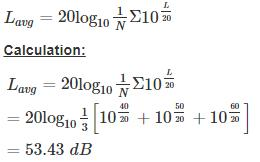Courses

# Test: Environmental Engineering- 6

## 10 Questions MCQ Test RRB JE for Civil Engineering | Test: Environmental Engineering- 6

Description
This mock test of Test: Environmental Engineering- 6 for Civil Engineering (CE) helps you for every Civil Engineering (CE) entrance exam. This contains 10 Multiple Choice Questions for Civil Engineering (CE) Test: Environmental Engineering- 6 (mcq) to study with solutions a complete question bank. The solved questions answers in this Test: Environmental Engineering- 6 quiz give you a good mix of easy questions and tough questions. Civil Engineering (CE) students definitely take this Test: Environmental Engineering- 6 exercise for a better result in the exam. You can find other Test: Environmental Engineering- 6 extra questions, long questions & short questions for Civil Engineering (CE) on EduRev as well by searching above.
QUESTION: 1

### Which kind of plume has minimum downward mixing as its downward motion is prevented by inversion and upward mixing will be quite turbulent and rapid?

Solution:

It is a condition where exists a strong super-adiabatic lapse rate above surface inversion.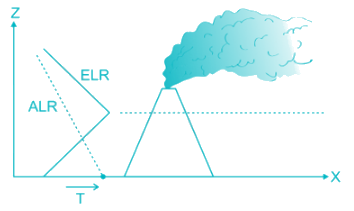Lofting plume has minimum downward mixing due to presence of inversion and is considered as ideal case for dispersion of emission.

QUESTION: 2

### According to the Noise Pollution (Regulation and Control) Rules, 2000, of the Ministry of Environment Forests, India, the day time and night time noise level limits in ambient air for residential area expressed in dB (A) Leq are

Solution:

Ambient air quality standards in respect of Noise as per Noise Pollution (Regulation and Control) Rules, 2000, of the Ministry of Environment & Forests, India are tabulated below: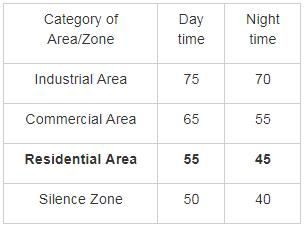QUESTION: 3

### Which of the following engineering system for air pollution control device can collect a particle size of less than one micrometer and can also have an efficiency as high as 99%

Solution:

The Particulate Control Devices description are as follow: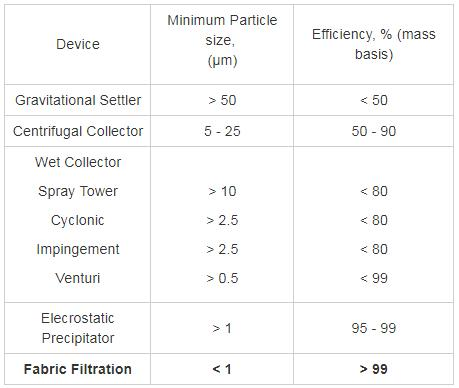Hence, Fabric Filtration can collect small particles as one micrometre and can also have an efficiency as high as 99% or more.

QUESTION: 4

Two primary air pollutants are

Solution:

Sulphur dioxide carbon monoxide, nitrogen oxides, lead, hydrocarbons, allergic agents like pollens and spores and radioactive substances are primary pollutants. Sulphuric acid, ozone, formaldehydes & peroxyacylnitrates (PAN) are secondary pollutants.

QUESTION: 5

An air parcel having 40° temperature moves from ground level to 500m elevation in dry air following the “adiabatic lapse rate”. The temperature of air parcel at 500 m elevation will be

Solution:

Dry air cools at the rate of 9.8°C per km and it is called dry adiabatic lapse rate. In saturated (wet) air, this rate is calculated to be 6°C per km and is known as wet adiabatic lapse rate.

Resulting temperature of air
= 40 − 9.8 × 500/1000 = 40 − 4.9 = 35.1C

QUESTION: 6

Arrange the layers of the atmosphere in order of increasing heights from the surface of the earth

Solution:

The Layers of atmosphere in order of increasing heights form the surge of earth

a) Troposphere_____ 0 to 11 km _____ 15 to -56°C

b) Stratosphere _____ 11 to 50 km _____ 15 to 0.2°C

c) Mesosphere _____ 50 to 85 km _____ -02° to -92°C

d) Thermosphere _____ 85 to 500 km _____ -92° to 1200°C

QUESTION: 7

Two electrostatic precipitators (ESPs) are in series. The fractional efficiencies of upstream and downstream ESPs for size dp are 80% and 65% respectively.

What is the overall efficiency of the system for the same dp?

Solution: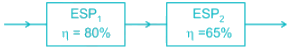Let X be the concentration of particles in flue gas.

∴ Particles remained after ESP1 = (1-0.8) X = 0.2 X

Particles remained after ESP2 = (1-0.65) 0.2 X = 0.07 X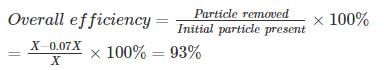QUESTION: 8

Assuming annual travel for each vehicle to be 50000 km, the quantity of NOx produced from 200,000 vehicle with emission rate 2.5 gm/km/vehicle is

Solution:

NOx emitted by 200,000 vehicles

= 2.5 × 200,000

= 500,000 g/km

NOx produced by 200,000 vehicles for a distance of 50000 km

= 500,000 × 50000 × 10-6 tonnes

= 25000 tonnes.

QUESTION: 9

The mean indoor airborne chloroform (CHCl3) concentration in a room was determined to be 0.4 µg/m3. Use the following data:

T = 293 K

P = 1 atmosphere

R = 82.05 × 10-6 atm.m3/mol-K

Atomic weights: C = 12, H = 1, Cl = 35.5. The concentration expressed in parts per billion (volume basis, ppbv) is equal to –

Solution: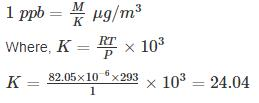Molecular weight, M = 12 + 1 + 3 × 35.5 = 119.5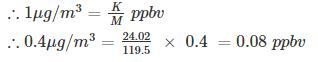QUESTION: 10

At a location three sound pressure levels were recorded as 40 dB, 50 dB, & 60 dB. The average sound pressure level is ________

Solution:

The average sound pressure level at that location is: